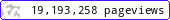•••••MadAsMaths.com :: I.Y.G.B. Practice Papers :: Undergraduate Methods Papers :: Mathematical Methods 1 Practice Papers

I.Y.G.B. MATHEMATICAL METHODS 1 PRACTICE PAPERS

These I.Y.G.B. practice papers follow the most common syllabi for a first year/first semester degree in Mathematics, Engineering or Science.

The examined topics are listed at the front cover of each paper.

All papers have model solutions.

 mathmeth1_a.pdfmathmeth1_a_solutions.pdfmathmeth1_b.pdfmathmeth1_b_solutions.pdfmathmeth1_c.pdfmathmeth1_c_solutions.pdfmathmeth1_d.pdfmathmeth1_d_solutions.pdfmathmeth1_e.pdfmathmeth1_e_solutions.pdfmathmeth1_f.pdfmathmeth1_f_solutions.pdfmathmeth1_g.pdfmathmeth1_g_solutions.pdfmathmeth1_h.pdfmathmeth1_h_solutions.pdfmathmeth1_i.pdfmathmeth1_i_solutions.pdfmathmeth1_j.pdfmathmeth1_j_solutions.pdfmathmeth1_k.pdfmathmeth1_k_solutions.pdfmathmeth1_l.pdfmathmeth1_l_solutions.pdfmathmeth1_m.pdfmathmeth1_m_solutions.pdfmathmeth1_n.pdfmathmeth1_n_solutions.pdfmathmeth1_o.pdfmathmeth1_o_solutions.pdfmathmeth1_p.pdfmathmeth1_p_solutions.pdfmathmeth1_q.pdfmathmeth1_q_solutions.pdfmathmeth1_r.pdfmathmeth1_r_solutions.pdfmathmeth1_s.pdfmathmeth1_s_solutions.pdfmathmeth1_t.pdfmathmeth1_t_solutions.pdfmathmeth1_u.pdfmathmeth1_u_solutions.pdfmathmeth1_v.pdfmathmeth1_v_solutions.pdfmathmeth1_w.pdfmathmeth1_w_solutions.pdfmathmeth1_x.pdfmathmeth1_x_solutions.pdfmathmeth1_y.pdfmathmeth1_y_solutions.pdfmathmeth1_z.pdfmathmeth1_z_solutions.pdf